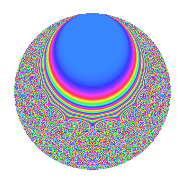# Properties

 Label 43.11.dLevel 43 Weight 11 Character orbit d Rep. character $$\chi_{43}(7,\cdot)$$ Character field $$\Q(\zeta_{6})$$ Dimension 72 Newform subspaces 1 Sturm bound 40 Trace bound 0

# Related objects

## Defining parameters

 Level: $$N$$ $$=$$ $$43$$ Weight: $$k$$ $$=$$ $$11$$ Character orbit: $$[\chi]$$ $$=$$ 43.d (of order $$6$$ and degree $$2$$) Character conductor: $$\operatorname{cond}(\chi)$$ $$=$$ $$43$$ Character field: $$\Q(\zeta_{6})$$ Newform subspaces: $$1$$ Sturm bound: $$40$$ Trace bound: $$0$$

## Dimensions

The following table gives the dimensions of various subspaces of $$M_{11}(43, [\chi])$$.

Total New Old
Modular forms 76 76 0
Cusp forms 72 72 0
Eisenstein series 4 4 0

## Trace form

 $$72q - 246q^{3} - 38122q^{4} - 3q^{5} + 8511q^{6} + 31377q^{7} + 675876q^{9} + O(q^{10})$$ $$72q - 246q^{3} - 38122q^{4} - 3q^{5} + 8511q^{6} + 31377q^{7} + 675876q^{9} - 127061q^{10} + 521524q^{11} - 606618q^{12} + 796742q^{13} - 865794q^{14} - 433513q^{15} + 22423366q^{16} - 1768471q^{17} - 11957547q^{18} - 122844q^{19} + 419841q^{20} - 26778860q^{21} + 16414886q^{23} + 8684578q^{24} + 70516989q^{25} + 3069q^{26} - 97624092q^{28} + 118701393q^{29} - 1455921q^{30} + 10824883q^{31} + 315141798q^{33} + 47529561q^{34} - 333788722q^{35} - 597728908q^{36} - 169881246q^{37} - 88887452q^{38} + 570788831q^{40} - 543049070q^{41} + 408159179q^{43} - 754130886q^{44} + 270181896q^{46} + 348527658q^{47} + 175039227q^{48} + 781091547q^{49} - 663244398q^{50} - 1853993086q^{52} + 219922736q^{53} + 3104867360q^{54} + 2154394182q^{55} + 2010912675q^{56} + 349107351q^{57} + 2023447828q^{58} + 2247595680q^{59} - 2906780465q^{60} + 508093974q^{61} - 3451752063q^{62} + 158921973q^{63} - 13473879716q^{64} + 1277847051q^{66} - 2812410281q^{67} + 13614613118q^{68} - 5113655829q^{69} + 2418191196q^{71} + 26243329290q^{72} - 6537901611q^{73} - 2632816966q^{74} - 10170994206q^{76} - 16936463889q^{77} - 24146069696q^{78} + 5397136602q^{79} + 7242149661q^{80} - 15745886176q^{81} + 4998656042q^{83} + 44726677232q^{84} - 27688564866q^{86} + 37895576172q^{87} - 12151857357q^{89} - 96024912764q^{90} + 36633770214q^{91} - 31643971023q^{92} + 59768940153q^{93} - 19802964089q^{95} - 3289705262q^{96} - 40329658574q^{97} + 19243888221q^{98} + 18506221111q^{99} + O(q^{100})$$

## Decomposition of $$S_{11}^{\mathrm{new}}(43, [\chi])$$ into newform subspaces

Label Dim. $$A$$ Field CM Traces $q$-expansion
$$a_2$$ $$a_3$$ $$a_5$$ $$a_7$$
43.11.d.a $$72$$ $$27.320$$ None $$0$$ $$-246$$ $$-3$$ $$31377$$

## Hecke characteristic polynomials

There are no characteristic polynomials of Hecke operators in the database